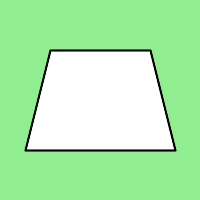Open In App

# Wand polygon() function in Python

polygon() function is another drawing function introduced in wand.drawing module. We can draw complex shapes using polygon() function. It takes a list of points in polygons as argument. Stroke line will automatically close between first & last point.

Syntax :

```wand.drawing.polygon(points)
```

Parameters :

ParameterInput TypeDescription
pointslistlist of x, y tuples.

Example #1

 `from` `wand.image ``import` `Image``from` `wand.drawing ``import` `Drawing``from` `wand.color ``import` `Color`` ` `with Drawing() as draw:``    ``draw.stroke_width ``=` `2``    ``draw.stroke_color ``=` `Color(``'black'``)``    ``draw.fill_color ``=` `Color(``'white'``)`` ` `    ``# points list for polygon``    ``points ``=` `[(``25``, ``25``), (``175``, ``100``), (``25``, ``175``)]`` ` `    ``# draw polygon using polygon() function``    ``draw.polygon(points)``    ``with Image(width ``=` `200``, height ``=` `200``, background ``=` `Color(``'lightgreen'``)) as image:``        ``draw(image)``        ``image.save(filename ``=` `"polygon.png"``)`

Output :Example #2:

 `from` `wand.image ``import` `Image``from` `wand.drawing ``import` `Drawing``from` `wand.color ``import` `Color`` ` `with Drawing() as draw:``    ``draw.stroke_width ``=` `2``    ``draw.stroke_color ``=` `Color(``'black'``)``    ``draw.fill_color ``=` `Color(``'white'``)`` ` `    ``# points list for polygon``    ``points ``=` `[(``50``, ``50``), (``150``, ``50``), (``175``, ``150``), (``25``, ``150``)]`` ` `    ``# draw polygon using polygon() function``    ``draw.polygon(points)``    ``with Image(width ``=` `200``, height ``=` `200``, background ``=` `Color(``'lightgreen'``)) as image:``        ``draw(image)``        ``image.save(filename ``=` `"polygon2.png"``)`

Output :